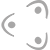#MLZ User Meeting 2021

Dec 7 – 8, 2021 Online only
Europe/Berlin timezone

## Superstructure in inverse perovskite nitrides

Only available for registered users!
Dec 8, 2021, 10:30 AM
1h 30m
Board: P-65
Poster Structure Research

### Description

We are investigating the crystal structure and properties of inverse perovskite nitrides with elpasolite-type superstructures. This superstructure is due to a full or partial ordering of nitrogen atoms and defects on the perovskite $B$ site. Since nitrogen scatters neutrons much more strongly than X-rays, neutron diffraction measurements of seven compounds with this superstructure were performed. Beforehand, we had illuminated their crystal structures using X-ray powder diffractometry, but were unable to determine the exact nature and extent of the superstructure.
After neutron diffraction measurements, the superstructures could be modeled much more reliably by simultaneous refinement of X-ray and neutron data, utilizing the advantages of both methods. For (Sr$_3$N$_{0.5}$)Ge, (Ba$_3$N$_{0.5}$)Ge, (Ba$_3$N$_{0.5}$)Sn and (Ba$_3$N$_{0.5}$)Pb, a full ordering of nitrogen and vacancies was confirmed. In contrast, analogous calcium compounds have a higher nitrogen content and show only partial ordering. One of the elpasolite B sites is fully occupied by nitrogen in every case. Most of the crystal structures feature octahedral tilting, which leads to an inverse Na$_3$[AlF$_6$]-type structure for (Ca$_3$N$_{0.69}$)Ge, (Sr$_3$N$_{0.5}$)Ge and (Ba$_3$N$_{0.5}$)Ge. (Ba$_3$N$_{0.5}$)Sn and (Ba$_3$N$_{0.5}$)Pb crystallize in space group $R\overline{3}$ with less pronounced tilting, while the inverse K$_2$(NaAlF$_6$)-type structure of (Ca$_3$N$_{0.65}$)Sn and (Ca$_3$N$_{0.56}$)Pb has no octahedral tilting.

### Co-authors

Rainer Niewa (Universität Stuttgart) Volodymyr Baran (DESY, FS-PETRA-D, P02.1) Dr Thomas Hansen (Institut Laue-Langevin Grenoble)

### Presentation materials

There are no materials yet.